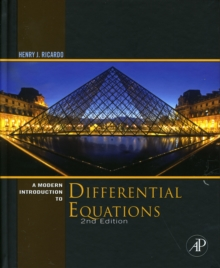# A MODERN INTRODUCTION TO DIFFERENTIAL EQUATIONS

by: Ricardo, Henry J.
Format: Hardcover

### price comparison#### Description

Product description A Modern Introduction to Differential Equations, Second Edition, provides an introduction to the basic concepts of differential equations. The book begins by introducing the basic concepts of differential equations, focusing on the analytical, graphical, and numerical aspects of first-order equations, including slope fields and phase lines. The discussions then cover methods of solving second-order homogeneous and nonhomogeneous linear equations with constant coefficients; systems of linear differential equations; the Laplace transform and its applications to the solution of differential equations and systems of differential equations; and systems of nonlinear equations. Each chapter concludes with a summary of the important concepts in the chapter. Figures and tables are provided within sections to help students visualize or summarize concepts. The book also includes examples and exercises drawn from biology, chemistry, and economics, as well as from traditional pure mathematics, physics, and engineering. This book is designed for undergraduate students majoring in mathematics, the natural sciences, and engineering. However, students in economics, business, and the social sciences with the necessary background will also find the text useful. Review An accessible introductory differential equations textbook for the one-semester course. About the Author Henry J. Ricardo works at Medgar Evers College of the City University of New York in Brooklyn, USA.

#### Details

Product Code: 9780123747464
ISBN: 0123747465
Publisher: Academic Press
Publication Date: 2009-03-26
Number of Pages: 536 pages
Languages: english
Edition: 2
Dimension: 7.72 x 1.1 x 9.29 inches
Shipping Weight: 2.25 pounds